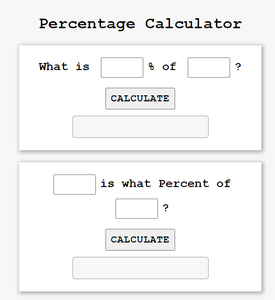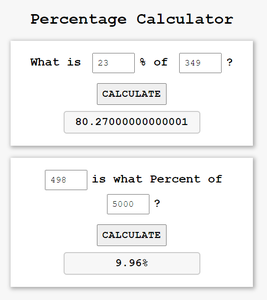Open in App
Not now

# Percentage calculator using HTML CSS and JavaScript

• Last Updated : 28 Jul, 2021

Do you want to calculate the percentage? If yes, then you can make a simple Percentage calculator using HTML, CSS, and JavaScript. In this article, you have to enter the marks obtained in the first input field and total marks in the second input field for calculating the percentage. After entering values and clicking on the Calculate button, a percentage can be obtained. This very useful project for calculating marks, discounts, etc. Percentage calculator is useful for students, shopkeepers, and for solving basic mathematical problems related to percentages.

File structure

• index.html
• style.css
• script.js

Prerequisites: Basic knowledge of HTML, CSS, and JavaScript needed. The project contains HTML, CSS, and JavaScript files. The HTML file adds structure, followed by styling using CSS and JavaScript adds functionality to it and for making validations to certain parts of the project.

HTML layout is created using the div tags, id attributes, classes, forms, and buttons for submission of the form. It defines the structure of the web page.

## index.html

 ```<``html` `lang``=``"en"``>`` ` `<``head``>``    ``<``meta` `charset``=``"UTF-8"``>``    ``<``meta` `http-equiv``=``"X-UA-Compatible"` `content``=``"IE=edge"``>``    ``<``meta` `name``=``"viewport"` `content``=``        ``"width=device-width, initial-scale=1.0"``>``    ``<``title``>Percentage Calculator``    ``<``link` `rel``=``"stylesheet"` `href``=``"style.css"` `type``=``"text/css"` `/>```` ` `<``body``>``    ``<``h1``>Percentage Calculator`` ` `    ``<``div``>`` ` `        ````        ``<``h2``> What is <``input` `type``=``"number"` `id``=``"percent"` `/>% of``            ``<``input` `type``=``"number"` `id``=``"num"` `/>?``        ```` ` `        ````        ``<``button` `onclick``=``"percentage_1()"``>Calculate<``br``>`` ` `            ````        ``<``input` `type``=``"text"` `id``=``"value1"` `readonly />``    ```` ` `    ``<``div``>``        ````        ``<``h2``><``input` `type``=``"number"` `id``=``"num1"` `/>``            ``is what Percent of``            ``<``input` `type``=``"number"` `id``=``"num2"` `/>?``        ````         ` `        ````        ``<``button` `onclick``=``"percentage_2()"``>Calculate``         ` `        ````        ``<``input` `type``=``"text"` `id``=``"value2"` `readonly />``    ````     ` `    ``<``script` `type``=``"text/javascript"` `src``=``"script.js"``>```` ` ``

By using CSS properties, we will decorate the web page using color, width, height, and position properties that are given as per the requirement in the project. CSS designs the interface of the calculator.

## style.css

 `/* A margin and padding are provided 0 ``box-sizing border box is used to include ``padding and border in the total width ``and height of the element, and font-family ``can be specified by the user */``* {``    ``margin``: ``0``;``    ``padding``: ``0``;``    ``box-sizing: border-box;``    ``font-family``: ``'Courier New'``, ``Courier``, ``monospace``;``}` `/* The user display allows you to specify the``background colour and height. The ``display:flex property, which is aligned at the``centre, is used to fill available free space ``or to shrink them to prevent overflow. */``body {``    ``background-color``: ``#f7f7f7``;``    ``min-height``: ``100``vh;``    ``display``: flex;``    ``justify-``content``: ``center``;``    ``align-items: ``center``;``    ``flex-``direction``: column;``}` `/* font-weight Specifies weight of glyphs ``in the font, their degree of blackness or ``stroke */``h``1` `{``    ``font-weight``: ``900``;``    ``margin-bottom``: ``12px``;``}` `div {``    ``width``: ``480px``;``    ``background-color``: ``#fff``;``    ``margin``: ``12px` `0``;``    ``padding``: ``24px``;``    ``text-align``: ``center``;``    ``box-shadow: ``2px` `2px` `8px` `2px` `#aaa``;``}` `input[type=number] {``    ``width``: ``84px``;``    ``font-size``: ``18px``;``    ``padding``: ``8px``;``    ``margin``: ``0px` `8px` `8px` `8px``;``}` `/* The text-transform:uppercase property ``causes characters to be raised to uppercase. ``The button's font-weight, font-size, and ``cursor type can be customised by the user. */``button {``    ``text-transform``: ``uppercase``;``    ``font-weight``: ``900``;``    ``font-size``: ``20px``;``    ``margin``: ``12px` `0``;``    ``padding``: ``8px``;``    ``cursor``: ``pointer``;``    ``letter-spacing``: ``1px``;``}` `/* The font-weight, font-size, background-color, ``and border can all be customized by the user. ``The border-radius property allows you to give ``an element rounded corners.*/``input[type=text] {``    ``font-size``: ``22px``;``    ``padding``: ``8px` `0``;``    ``font-weight``: ``900``;``    ``text-align``: ``center``;``    ``background-color``: ``#f7f7f7``;``    ``border``: ``2px` `solid` `#ccc``;``    ``border-radius: ``6px``;``}`

To add functionality to a web page, JavaScript code is used. In this case, two functions, percentage 1() and percentage 2(), are declared and passed to the button via the onclick event. As a result, when the Calculate button is pressed, the result is displayed. We used the method document.getElementById(). It returns the element with the specified ID attribute and returns null if no elements with the specified ID exist.

Formula used:

What is X percent of Y is given by the formula:
X percent of Y =(X/100)* Y

And X is what percent of Y is given by the formula:
X is what percent of Y= (X/Y)×100%, where X, Y > 0

## script.js

 `function` `percentage_1() {`` ` `    ``// Method returns the element of percent id``    ``var` `percent = document.getElementById(``"percent"``).value;``     ` `    ``// Method returns the element of num id``    ``var` `num = document.getElementById(``"num"``).value;``    ``document.getElementById(``"value1"``)``        ``.value = (num / 100) * percent;``}`` ` `function` `percentage_2() {`` ` `    ``// Method returns the element of num1 id``    ``var` `num1 = document.getElementById(``"num1"``).value;``     ` `    ``// Method returns the elements of num2 id``    ``var` `num2 = document.getElementById(``"num2"``).value;``    ``document.getElementById(``"value2"``)``        ``.value = (num1 * 100) / num2 + ``"%"``;``}`

#### Output:

• At First-Percentage Calculator

• After entering some data and Clicking on Calculate Button-Percentage Calculator

My Personal Notes arrow_drop_up